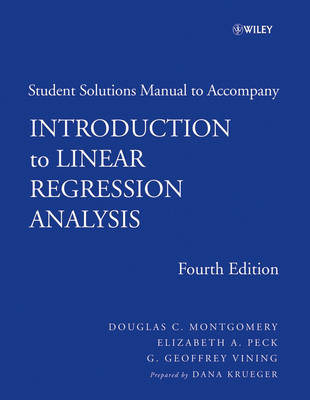•# Introduction to Linear Regression Analysis: Student Solutions Manual (Paperback)

(author), (author), (author)
£24.95
Paperback Published: 27/03/2007

This product is currently unavailable.

A comprehensive and up-to-date introduction to the fundamentals of regression analysis The Fourth Edition of Introduction to Linear Regression Analysis describes both the conventional and less common uses of linear regression in the practical context of today's mathematical and scientific research. This popular book blends both theory and application to equip the reader with an understanding of the basic principles necessary to apply regression model-building techniques in a wide variety of application environments. It assumes a working knowledge of basic statistics and a familiarity with hypothesis testing and confidence intervals, as well as the normal, t, x2, and F distributions. Illustrating all of the major procedures employed by the contemporary software packages MINITAB(r), SAS(r), and S-PLUS(r), the Fourth Edition begins with a general introduction to regression modeling, including typical applications. A host of technical tools are outlined, such as basic inference procedures, introductory aspects of model adequacy checking, and polynomial regression models and their variations. The book discusses how transformations and weighted least squares can be used to resolve problems of model inadequacy and also how to deal with influential observations. Subsequent chapters discuss: Indicator variables and the connection between regression and analysis-of-variance models Variable selection and model-building techniques and strategies The multicollinearity problem--its sources, effects, diagnostics, and remedial measures Robust regression techniques such as M-estimators, and properties of robust estimators The basics of nonlinear regression Generalized linear models Using SAS(r) for regression problems This book is a robust resource that offers solid methodology for statistical practitioners and professionals in the fields of engineering, physical and chemical sciences, economics, management, life and biological sciences, and the social sciences. Both the accompanying FTP site, which contains data sets, extensive problem solutions, software hints, and PowerPoint(r) slides, as well as the book's revised presentation of topics in increasing order of complexity, facilitate its use in a classroom setting. With its new exercises and structure, this book is highly recommended for upper-undergraduate and beginning graduate students in mathematics, engineering, and natural sciences. Scientists and engineers will find the book to be an excellent choice for reference and self-study.

Publisher: John Wiley and Sons Ltd
ISBN: 9780470125069
Weight: 366 g
Dimensions: 280 x 219 x 10 mm
Edition: 4th Revised edition